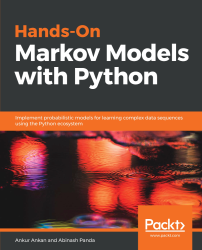•#### Hands-On Markov Models with Python#### Overview of this book

Hidden Markov Model (HMM) is a statistical model based on the Markov chain concept. Hands-On Markov Models with Python helps you get to grips with HMMs and different inference algorithms by working on real-world problems. The hands-on examples explored in the book help you simplify the process flow in machine learning by using Markov model concepts, thereby making it accessible to everyone. Once you’ve covered the basic concepts of Markov chains, you’ll get insights into Markov processes, models, and types with the help of practical examples. After grasping these fundamentals, you’ll move on to learning about the different algorithms used in inferences and applying them in state and parameter inference. In addition to this, you’ll explore the Bayesian approach of inference and learn how to apply it in HMMs. In further chapters, you’ll discover how to use HMMs in time series analysis and natural language processing (NLP) using Python. You’ll also learn to apply HMM to image processing using 2D-HMM to segment images. Finally, you’ll understand how to apply HMM for reinforcement learning (RL) with the help of Q-Learning, and use this technique for single-stock and multi-stock algorithmic trading. By the end of this book, you will have grasped how to build your own Markov and hidden Markov models on complex datasets in order to apply them to projects.
PrefaceFree Chapter
Introduction to the Markov ProcessHidden Markov ModelsState Inference - Predicting the StatesParameter Learning Using Maximum LikelihoodParameter Inference Using the Bayesian ApproachTime Series PredictingNatural Language Processing2D HMM for Image ProcessingMarkov Decision ProcessOther Books You May Enjoy# The Markov reward process

In the previous section, we gave an introduction to MDP. In this section, we will define the problem statement formally and see the algorithms for solving it.

An MDP is used to define the environment in reinforcement learning and almost all reinforcement learning problems can be defined using an MDP.

For understanding MDPs we need to use the concept of the Markov reward process (MRP). An MRP is a stochastic process which extends a Markov chain by adding a reward rate to each state. We can also define an additional variable to keep a track of the accumulated reward over time. Formally, an MRP is defined bywhere S is a finite state space, P is the state transition probability function, R is a reward function, andis the discount rate:wheredenotes the expectation. And the term Rs here denotes the expected reward at the state s.

In the case of...